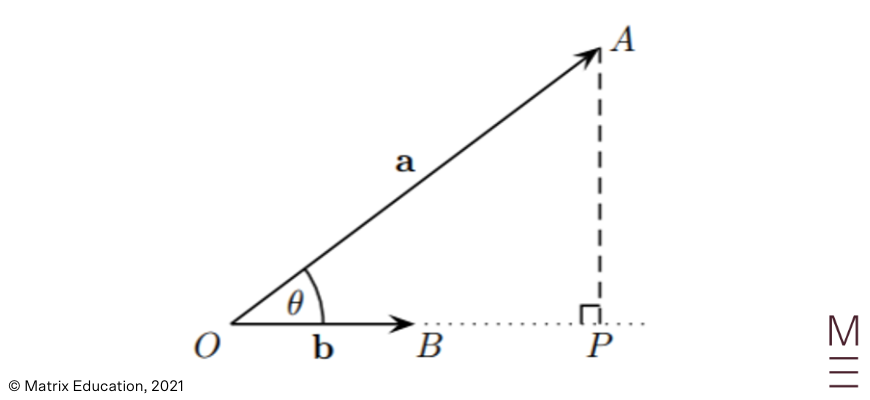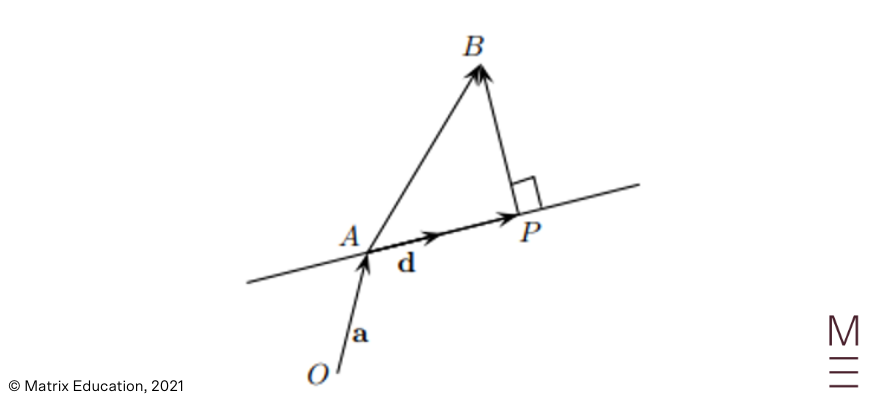# Part 3: Vector Projections | Beginner’s Guide to Year 12 Maths Ext 1

Ace your next vector projections test now!Still confused about vector projections? Well, you don’t need to be! In this article, we’ll go through everything you need to know about vector projections.

• The NESA Syllabus Outcomes
• Vectors Projections
• Applications of Projection
• Concept Check Questions

## Year 12 Mathematics Extension 1: Vector Projections

Within the topic of vectors there is a specific requirement for students to be familiar with the concept of the projection of one vector onto another.

This syllabus dot point allows for students to develop their understanding of vector operations and can be used in applications to both harder exam questions and real world problems.

## NESA Syllabus Outcomes

• Define and use the projection of one vector onto another

## Assumed Knowledge

Students should have a basic understanding of vectors, as well as some fundamental properties and operations including the dot product. Students should also feel comfortable with concepts of trigonometry and coordinate geometry.

This content can be found in our previous mathematics guides should students want to solidify their understanding.

## Vector Projection

The idea of a vector projection, in its simplest form is just the question of how much one vector goes in the direction of another. This idea is geometrically represented by the figure below, with vector a being projected onto vector $$b$$.

The projection in this case would be the vector $$OP$$. We can see that $$OP$$ is parallel to vector $$b$$.We can also see from the triangle that the following relationship should hold:

\begin{align*}
cos⁡( \theta ) = \frac{OP}{a}
\end{align*}

Hence,

\begin{align*}
OP|=|a|\times |cos⁡( \theta )|
\end{align*}

Using our definition of the dot product as $$a \cdot b =|a|\times |b|\times cos \theta$$, we can rewrite the above expression:

\begin{align*}
|OP|= \frac{|a \cdot b|}{|b|}
\end{align*}

From here, we can use the idea that the unit vector $$\frac{b}{|b|}$$ is simply a vector with unit length pointing in the direction of vector $$b$$ to formally define a formula for the vector projection in terms of the dot product.

For vectors $$a, \ b \ \in \ R^n$$ and $$b \neq 0$$, the projection of a onto b is given by:

\begin{align*}
proj_{b} a = \big{(} \frac{a \cdot b}{|b|^2 } \big{)} b
\end{align*}

## Scalar Projection

The scalar projection is essentially derived from the concept of a vector projection. The only difference between the two is that the scalar projection, as implied in its name is simply a scalar measure of the length of the projection created from one vector onto another.

For vectors $$a, \ b \ \in \ R^n$$ and $$b \neq 0$$, the scalar projection of $$a$$ onto $$b$$ is given by:

\begin{align*}
| proj_{b} a | = \big{(} \frac{ |a \cdot b |}{|b| } \big{)}
\end{align*}

We can observe that the scalar projection is simply the expression for the vector projection with the removal of the unit vector $$\frac{b}{|b|}$$.

This can be attributed to the scalar projection being a scalar value, hence the removal of the directional vector component of the vector projection should simply leave us with a measurement of the projection’s length.

## Ace Vector Projections with these practice questions!## Applications to Problems

Now that we understand the concept of projections, let’s try applying this idea to some examples:

### Example 1

Find the distance from the point $$(2, -1, 3)$$ to the line through the points $$(0, 1, 4)$$ and $$(4, 2, 9)$$

### Solution 1

At first glance, it might not be obvious that the idea of vector projection can be used in solving this question. However, recall that the distance between a point and a line is simply the perpendicular distance taken from the base of the line to the point, similar to the formula taught in year 10.

A good place to start for this question would be to label each point. Hence let $$A, \ B$$ and $$C$$ be the points $$(0, 1, 4), (2, -1, 3)$$ and $$(4, 2, 9)$$ respectively. This subsequently implies the vectors $$AB$$ and $$AC$$ are represented by $$(2, -2, -1)$$ and $$(4, 1, 5)$$ respectively.

We can see in the previous diagram that a vector projection essentially creates a perpendicular from the end of the projecting vector to the base of the vector being projected upon.

If we let $$P$$ denote the base of said perpendicular, we can see that the perpendicular distance between the point and the line is simply the distance $$BP$$.By taking the scalar projection of the vector $$AB$$ onto the vector $$AC$$ :

\begin{align*}
| proj_{AC} AB | = \frac{|AB \cdot AC|}{|AC|} =\frac{ |8-2-5|}{ \sqrt{16+1+25}} = \frac{1}{\sqrt{42}}
\end{align*}

We have now found the length of the vector $$AP$$. With reference to our diagram, we can see that to find the length of the vector $$BP$$, we can just apply Pythagoras theorem as it’s relatively simple to determine the length of the vector $$AB$$:

\begin{align*}
|AB|= \sqrt{4+4+1} =3
\end{align*}

Hence, the distance from the point to the line is given by:

\begin{align*}
| BP| = \sqrt{3^2 – \frac{1}{42}} = \frac{ \sqrt{377}}{\sqrt{42}}
\end{align*}

### Example 2

Let $$u$$  and $$v$$  be non-zero vectors such that $$|u|=|v|$$. Show that $$| proj_v \ u |= |proj_u \ v |$$

### Solution 2

For proof questions such as this one, it can be a good idea to first convert functions or operations into simpler terms. In this case, let’s begin the question by writing out the formula for vector projection:

\begin{align*}
LHS= \bigg{|} \bigg{(} \frac{u \cdot v}{|v|^2} \bigg{)} v \bigg{|} \\
RHS= \bigg{|} \bigg{(} \frac{v \cdot u}{|u|^2} \bigg{)} u \bigg{|}
\end{align*}

Now that we’ve done that, it can be beneficial to also extract all the information provided to us in the question. In this case, the only condition we’re given is that $$|u|=|v|$$. Let’s try to manipulate the LHS with this condition to try and make it resemble the RHS a bit more:

\begin{align*}
LHS= \bigg{|} \bigg{(} \frac{u \cdot v}{|v|^2} \bigg{)} v \bigg{|}
\end{align*}

Since $$|u|=|v|$$, it should follow that $$|u|^2 =|v|^2$$. Hence we can rewrite the LHS:

\begin{align*}
LHS= \bigg{|} \bigg{(} \frac{u \cdot v}{|u|^2} \bigg{)} v \bigg{|}
\end{align*}

We can also use the property that $$u \cdot v = v \cdot u$$ to further manipulate the expression:

\begin{align*}
LHS= \bigg{|} \bigg{(} \frac{v \cdot u}{|u|^2} \bigg{)} v \bigg{|}
\end{align*}

From here, it can be a little difficult to see that we’ve essentially finished the proof already. It’s important for students to make the observation that $$\big{(} \frac{ v \cdot u}{|u|^2 } \big{)}$$ is simply a scalar value, meaning that it can be separated from the vector component of the expression.

\begin{align*}
LHS= \bigg{|} \bigg{(} \frac{v \cdot u}{|u|^2} \bigg{)} \bigg{|} \times | v|
\end{align*}

Now using the given condition that $$|u|=|v|$$:

\begin{align*}
LHS &=\bigg{|} \bigg{(} \frac{v \cdot u}{|u|^2 } \bigg{)} \bigg{|} \times |u| \\
&= \bigg{|} \bigg{(} \frac{v \cdot u}{|u|^2 } \bigg{)} u \bigg{|}\\
&=RHS \\
∴|proj_v \ u|&= |proj_u \ v|
\end{align*}

The proof is complete.

## Concept Questions

1. Find the dot product of vectors $$a=(-4,1)$$ and $$b=(1,2)$$

2. Sketch the vectors $$a=(-4,1)$$ and $$b=(1,2)$$

3. Find the vector resulting from the projection of $$a=(-4,1)$$ onto $$b=(1,2)$$

4. Find the length of the projection of $$(-4, 1)$$ onto $$(1, 2)$$

1. $$a \cdot b=-2$$

2. Diagram

3. $$\big{(} \frac{8}{17}, \ – \frac{2}{17} \big{)}$$

4. $$\frac{2}{\sqrt{17}}$$

## Save time and boost your marks

Our HSC experts will break down Maths concepts through our structured video lessons, and our comprehensive resources will give you plenty of practice to ace your exams. Learn more now.© Matrix Education and www.matrix.edu.au, 2021. Unauthorised use and/or duplication of this material without express and written permission from this site’s author and/or owner is strictly prohibited. Excerpts and links may be used, provided that full and clear credit is given to Matrix Education and www.matrix.edu.au with appropriate and specific direction to the original content.